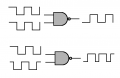# Frequency of NAND gate output signal

#### anhnha

Joined Apr 19, 2012
901
I have to square wave signals with the same frequency where one is delayed compared to the other.
Put two signals to the input of a NAND gate (two inputs).
Is there way (maybe math) to see that the output signal of NAND gate also has the same frequency as the input signal?

#### Dodgydave

Joined Jun 22, 2012
10,829
You need an Oscilloscope to view the output, note a Nand gate will only go low when both inputs are high.

#### dannyf

Joined Sep 13, 2015
2,197
Unless the two signals are exactly out of phase, the output has the same frequency as the input but the duty cycle reflects the phase differential.

#### anhnha

Joined Apr 19, 2012
901
Well, is the a way to proof that in theory?

#### Tonyr1084

Joined Sep 24, 2015
7,356
Assume for a moment that you're feeding the square wave input to BOTH inputs of the 2 input NAND gate. Being a "NAND" it will have the exact same frequency as the input BUT at a phase shift of 180°. No need for math. Whatever the input frequency is the output frequency would be identical (with the exception of being out of phase).

In your question you state clearly that the second input is "the same frequency but delayed", out of phase with the first. The output frequency will still be exactly the same, only the duty cycle will vary. IF the second input frequency was delayed by 90° then the output would have a duty cycle of 25% high, 75% low. The frequency will remain the same, only the period in which the square wave (which is not symmetrically square) will change.

I'll draw a picture and post it shortly.

Below is a 2 in NAND gate. The square waves on the upper gate are in synchronous time. The output will be the exact opposite of the two synchronized inputs (as can be seen by the wave form on the output.

In the lower gate you see the two square waves have been shifted a little. Since you didn't specify how much delay I can't draw you an exact picture. Nevertheless, notice that the output is high most of the time. The ONLY time it goes low is when both inputs are high at the same time. No matter which way you shift the input waveforms you will get either an equal time high or a nearly always high. Remember, it takes two high inputs to get a low output. IF you use an AND gate then the signal will be low more of the time.Last edited:
•anhnha

#### anhnha

Joined Apr 19, 2012
901
Thank you. I've drawn some cases but I don't believe in myself!

#### anhnha

Joined Apr 19, 2012
901
Thank you for the help and nice pictures.

#### Tonyr1084

Joined Sep 24, 2015
7,356

The first drawing posted is slightly flawed. I did the second drawing to clarify. Then I edited it for greater clarity. Then I edited it again. And again. OK, I'm done now. I hope finally all things are clear. I tend to make more mess than I clean up sometimes.

If you have any further questions feel free to post them here. Someone will answer you, and probably clearer on the first go around.

•anhnha

#### anhnha

Joined Apr 19, 2012
901
Yes. The second drawing has time scale so it is easier to see the result. Thank you again!

#### AnalogKid

Joined Aug 1, 2013
10,302
Is there way (maybe math) to see that the output signal of NAND gate also has the same frequency as the input signal?
Yes.

A .AND. B = C (don't know how to put in the symbol for a logical AND)

Thanks to Leibniz, Boole, and De Morgan, there are algebraic expressions for the basic logic gates that define their operation.

The truth table in post #6 is the answer. By the definition of what a NAND gate is, all four states will occur once each during each cycle. The exception noted in post #3 is possible in theory only at quantum-level timing. In practice with real-world parts it is slightly more possible to have an effective "tie" at the inputs and produce no output change, but the statement of the problem in post #1 eliminates this input condition.

ak

Last edited:

#### hp1729

Joined Nov 23, 2015
2,304
I have to square wave signals with the same frequency where one is delayed compared to the other.
Put two signals to the input of a NAND gate (two inputs).
Is there way (maybe math) to see that the output signal of NAND gate also has the same frequency as the input signal?
What math is needed? How can it be otherwise?